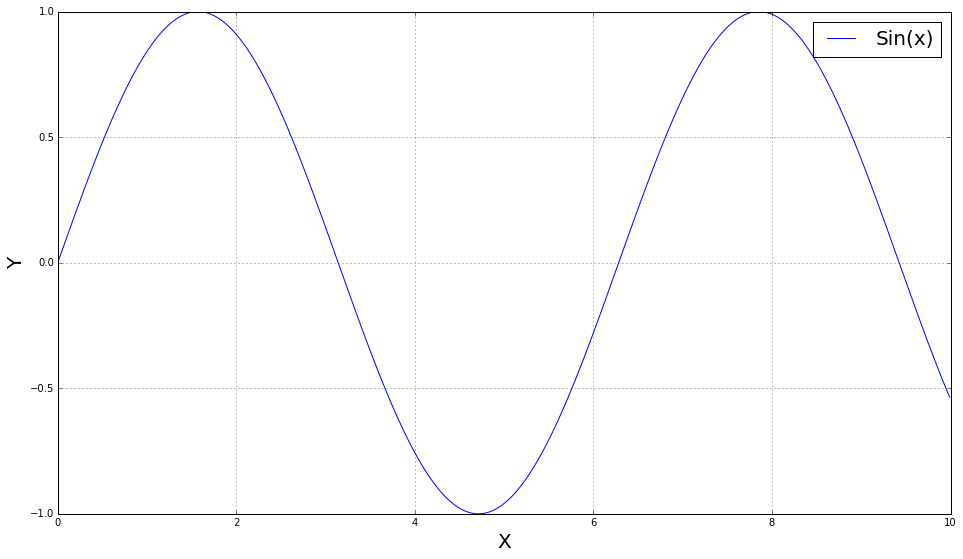# Tutorial 00: jupyter basics

Posted on Wed 06 December 2017 in misc by Corey Adams, Kazuhiro Terao

This post covers the very basics of how to use a Jupyter notebook. If you're already somewhat familiar with it, you can check this link for more comprehensive guide.

The name "notebook" comes from the fact that it literally acts like a notebook where you write down your findings, formulas, etc.. It's awesome because you can "execute code" you wrote, or keep texts as just notes, "make comments", and "keep figures in place". Let's explore these basics.

## Python code¶

The individual blocks of notebook interface are called cells, and you use shift+enter to execute a cell. The actions taken persist in backend of Jupyter called the kernel. By default, these blocks are executed as a python code (by a python kernel).

In :
from __future__ import print_function
x = 5

In :
print(x)

5


The order in which cells execute matters, so if you update a variable in a later cell, and reexecute a previous one, results can change. The order of execution can be seein inside the square bracket on the left of executed code block.

You can comment your python block as you would normally do:

In :
# This is a python code block


... but that looks not great (especially in this color scheme!). Another way to comment is this, how I have been writing this notebook as a Markdown cell. You can also use

HTML syntax with your custom CSS
to display something more elaborate like rainbow colored texts :)

## Plotting a figure¶

You can import anything just as you would normally do in your python session

In :
import numpy
import matplotlib.pyplot as plt
%matplotlib inline


The last line above tells jupyter to show all matplotlib plots below the code that created them, and if you save the notebook and commit it to, say, github then the plots will be shown in the browser when looking at the notebook:

In :
x = numpy.arange(0,10, 0.01)
y = numpy.sin(x)
fig, ax = plt.subplots(figsize=(16,9))

plt.plot(x, y, label="Sin(x)")
plt.grid(True)

plt.xlabel("X", fontsize=20)
plt.ylabel("Y", fontsize=20)
plt.legend(fontsize=20)
plt.show()Once you got these basics (and play a bit more by yourself), again I advertise this awesome notebook guidance. The community of Jupyter notebook is huge, so you will find answers to most of your questions, if not all, from professor Google.